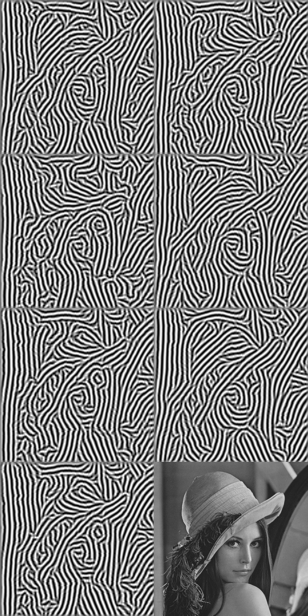Categories

# Image Orientation

I want to get a sense of the local angle of features in an image. This is a tall order at times, and isn’t even clearly defined. Do you want to follow the local gradient of the image? Be parallel to lines? We’ll try various methods and see what works. I’ll show a comparison of different methods at the bottom.

Simplest method, figure out the angle of the gradients of the image. Call the image $I(x,y)$, and its gradients are $\frac{dI}{dx} = I_x, \, \frac{dI}{dy}= I_y$ . the orientation $\theta$ is $\tan(\theta_{grad}) = I_y/I_x$. Depending on how the gradients are computed, and how smooth the image is, this might turn out to be effective or terrible. If the image isn’t smooth, they’ll be noisy, giving bad orientations.

The simplest way to estimate the gradients is via finite (forward) difference: $I_x(x,y) \approx (I(x+\Delta x,y)-I(x,y))/\Delta x$. A better method is central difference, $I_x(x,y) \approx (I(x+\Delta x,y)-I(x-\Delta x,y))/(2\Delta x)$. Unfortunately, if the image is noisy, this leads to very noisy (bad) estimates of the gradients. One way to combat this is with filtering.

The simplest filter is to just locally average the image, yielding $\overline{I}(x,y)$. Then take the gradients of this smoothed image to find $\tan(\theta_{smoothImage}) = \overline{I_y}/ \overline{I_x}$. This works decently, but blurs sharp edges.

Another option is to try to average the angles themselves, $\tan(\theta_{smoothAngle}) = \overline{\sin(\theta_0)} / \overline{ \cos(\theta_0) }$. Or a better approach is to do a bit of calculus to find the angle $\theta$ that is most aligned other local angles. Say that the cost function that we want to maximize is:

\begin{aligned} F(\theta) &= \sum_i^N \frac{1}{N} \left( \cos(\theta_i)\cos(\theta) + \sin(\theta_i)\sin(\theta) \right)^2 \\ &= \cos(\theta)^2 \, \overline{\cos(\theta_i)^2} + \sin(\theta)^2 \, \overline{ \sin(\theta_i)^2 } + \cos(\theta) \sin(\theta) 2 \, \overline{ \cos(\theta_i) \sin(\theta_i) } \end{aligned}

And maximizing that yields

\begin{aligned} \frac{d F}{d \theta} =0 &= ( \overline{ \sin(\theta_i)^2 } - \overline{\cos(\theta_i)^2} ) \sin(2 \theta) + 2 \, \overline{ \cos(\theta_i) \sin(\theta_i) } \cos(2 \theta) \\ \tan(2\theta _{aligned} ) &= \frac{ 2 \, \overline{ \cos(\theta_i) \sin(\theta_i) } }{ \overline{ \sin(\theta_i)^2 } - \overline{\cos(\theta_i)^2} } \end{aligned}.

The previous methods all provided an angle between $\pm \pi$, but this one yields an angle between $\pm \pi/2$. This is still useful, because sometimes you don’t care whether the orientation field points uphill or downhill, as long as they’re parallel.

## Least-square fit

Instead of computing the gradients via finite difference, Savitsky-Golay filtering give a nice way to find the local average gradient (with a least-squares fit) via convolution. This is much less susceptible to noise than simple finite difference. This gives $\tan(\theta_{SG}) = I_{y,SG} / I_{x,SG}$.

## Eigenvalues

Another method is based on the eigenvectors of the 2D structure tensor (or gradient-square tensor). These eigenvectors yield the directions along which the gradients change the most.

\begin{aligned} \lambda_1 &= \frac{1}{2} ( \overline{I_x^2} + \overline{I_y^2} ) + \frac{1}{2} \sqrt{\left( \overline{I_x^2} - \overline{I_y^2} \right)^2 + 4 \overline{I_x I_y}^2 } \\ \lambda_2 &= \frac{1}{2} ( \overline{I_x^2} + \overline{I_y^2} ) - \frac{1}{2} \sqrt{\left( \overline{I_x^2} - \overline{I_y^2} \right)^2 + 4 \overline{I_x I_y}^2 } \\ \tan(\theta) &= \frac{\lambda_1- \overline{I_x^2}}{ \overline{I_x I_y} } \end{aligned}

This turns out to be nearly equivalent to the calculus-based approach.

## Edge Tangent Flow

ETF is great for cleaning up an orientation field. It retains edges well, and gets rid of little annoying sections of directions.

## Comparison

The following image shows these orientation techniques applied to the Lena image. On the left is the angle from 0 to 360 degrees, and the right is wrapped around from 0 to 180 degrees. The color scheme is such that red is both 0 degrees and the maximum, wrapping around.

The gradient image has a lot of detail, and also substantial noise due to taking gradients of a natural image. The smoothing techniques remove much of the noise, but blur the edges. The optimal-alignment and eigenvalues-based techniques do a good job giving large swaths of smooth angles.Different orientation methods. From top to bottom: (1) central difference, (2) gradient of smoothed image, (3) smoothed angles, (4) optimal alignment, (5) Savitsky-Golay, (6) eigenvalues of structure matrix, (7) ETF

What’s the take-away? Don’t use central difference, since it’s too noisy. If you want the full-range angle, use Savitzky-Golay and clean it up with Edge Tangent Flow. If you want half-angles, use the optimal-alignment approach. Each of these approaches require some fine-tuning of the filtering radius, depending on how detailed you want it.

It’s hard to properly visualize the orientations just from those colored images, so here are stripes generated from those:Row (1) central difference and gradient of smoothed image; (2) smoothed angles and optimal alignment; (3) Savitsky-Golay and eigenvalues of structure matrix; (7) ETF and original image

The best approaches (with long continuous lines) seem to be the optimal alignment and eigenvalues. The smoothed image works decently, but loses some of its edges. ETF doesn’t rely on the edges as much, but is still nice.

This site uses Akismet to reduce spam. Learn how your comment data is processed.